## General Math Quiz

[qwiz style = “border: 2px solid black; background: white;"] [i] Test your knowledge on general math topics! Click start to begin! [q] Find the value of t in parallelogram DEFG.
(Hint: In a parallelogram opposite sides are congruent)

t = [textentry suggest = "false"]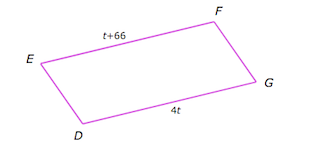[c*] 22 [q] X and U are the midpoints of the legs, SW and TV of trapezium STVW
If UX = 67 and ST = 89, what is VW?

VW = [textentry suggest = "false"]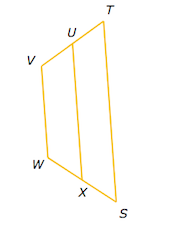[c*] 45 [q] Plot the graph that represents 10x + 5y > -4. Then see if you got it right. [c] Show the answer [f]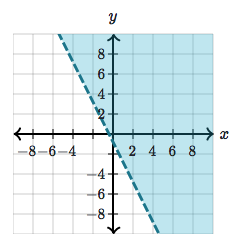[q] Find q
(Hint: Recall the Law of Sines)

q = [textentry suggest = "false"]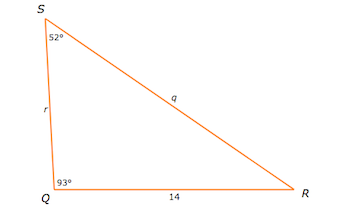[c*] 17.7 [q] Find WY
(Hint: Notice this is a right triangle)

WY = [textentry suggest = "false"]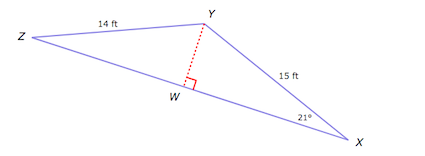[c*]5.4 [q] Quadrilateral WXYZ is a kite.
What is Y?

[textentry suggest = "false"] degrees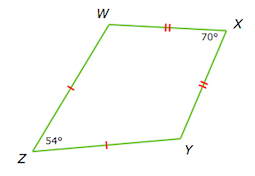[c*] 118 [q] Who discovered the Theory of Relativity?
[textentry suggest=”false”]   [c*] Albert Einstein [c*] albert einstein [c*] Einstein [c*] einstein [/qwiz]